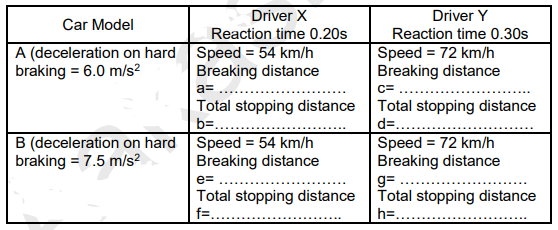Deepak Scored 45->99%ile with Bounce Back Crack Course. You can do it too!

# Complete the following table:

Question:

Complete the following table:Solution:

If initial velocity is $\mathrm{u}$ and deacceleration is $-\mathrm{a}$ then braking distance

$v^{2}=u^{2}+2 a s$

$0^{2}=u^{2}-2 a S$

$\mathrm{S}_{\mathrm{b}}=\frac{\mathrm{u} 2}{2 \mathrm{a}}$ (Braking distance)

$\mathrm{S}_{\mathrm{R}}=\mathrm{u} \times \mathrm{t}_{\mathrm{R}}$ (Reaction distance)

Total distance $=\mathrm{S}_{\mathrm{b}}+\mathrm{S}_{\mathrm{R}}$

Solve table with given values and above formulas